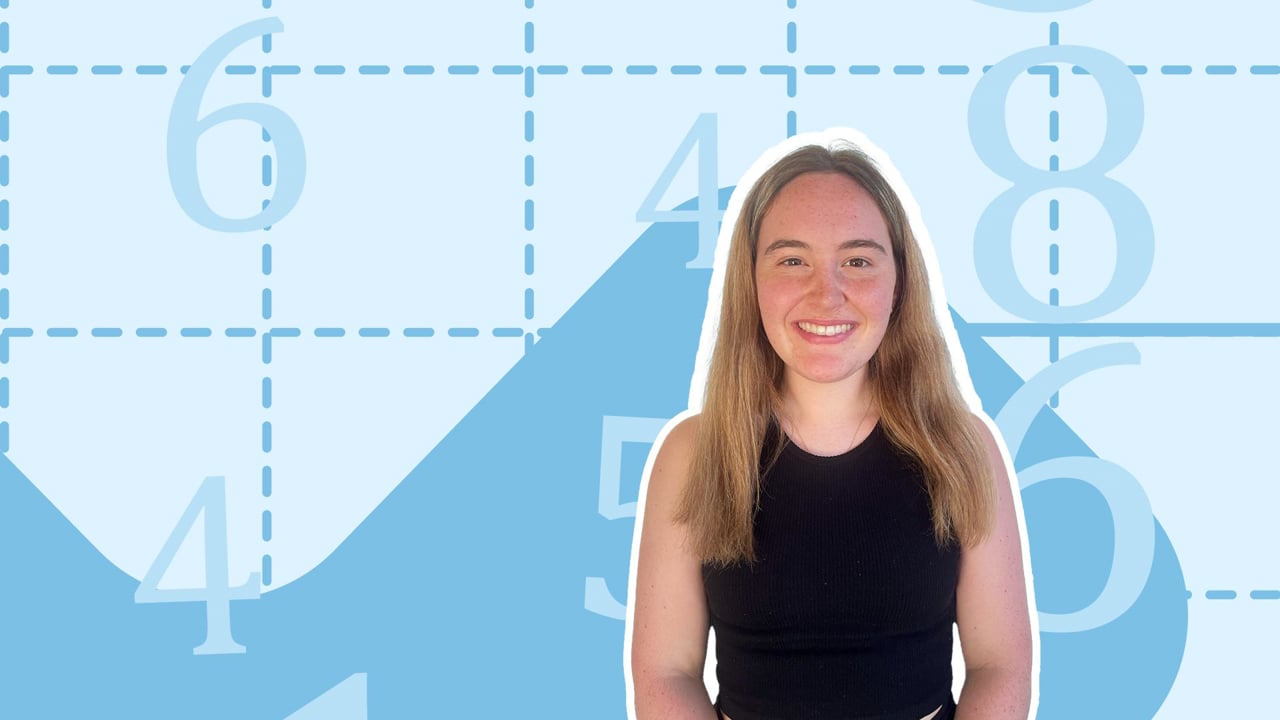Chapter overviewMaths

Exam board

AQA

Number

Algebra

Graphs

Ratio proportion and rates of change

Shapes and area

Angles and geometry

Trigonometry

Probability

Statistics

Maths

# Finding percentages and percentage change0%

Summary

# Finding percentages and percentage change

## ​​In a nutshell

Percentages are proportions out of one hundred and are commonly used to describe changes between numbers. They compare how much things such as value have grown or deteriorated over time.

## Finding percentages

There are a few different ways you might be asked to find a percentage - it's important to remember that a percentage can be converted to a fraction or a decimal to make things easier.

### Finding a percentage of a value - calculator method

To find a percentage of a value using a calculator, convert the percentage to a fraction and multiply it by the value.

##### Example 1

Find $19\%$ of $56.$ You may use a calculator for this question.

Convert to a fraction.

$19\%=\frac{19}{100}$​​

Multiply by the value.

$\frac{19}{100}\times56=\underline{10.64}$

### Finding a percentage of a value - non-calculator method

To find a percentage of a value without a calculator, split up the percentage into simple percentages, work each one out and add them together.

##### Example 2

Find $22\%$ of $89$. Do not use a calculator for this question.

Break down $22\%$ into simple percentages.

$22\%=10\%+10\%+1\%+1\%$

Work out each one.

$10\% = 89\div10=8.9\\1\%=89\div100=0.89$

$8.9+8.9+0.89+0.89=\underline{19.58}$​​

### Expressing one value as a percentage of the other

Or you may be asked to express one value as a percentage of another. Simply write the values as a fraction and multiply by one hundred.

##### Example 3

Express $93$​ as a percentage of $600$.

Write as a fraction.

$\frac{93}{600}$​​

Multiply by one hundred.

$\frac{93}{600}\times100 = 15.5$

As a percentage, $93$ is $\underline{15.5\%}$ of $600$.​

## Percentage changes

Percentage changes are calculated using multipliers and result in either a decrease on an increase.

### Definition

 Multiplier A decimal value that represents a percentage change:A % increase is represented by a multiplier greater than one.A % decrease is represented by a multiplier less than one.

To find a multiplier add or subtract your percentage as a decimal from one.

### Using percentage changes

To work out a change in percentage, calculate your multiplier and multiply by the original value.

##### Example 4

What is $£20$​ after a $17\%$ decrease?

Work out the multiplier: we have a decrease so take $0.17$ away from one.

$1-0.17=0.83$​​

Multiply the original value by the multiplier.

### $£20\times0.83=\underline{£16.60}$​

Tip: Simple interest involves making the an increase and then multiplying by the number of years.

### ​​Finding the percentage change

In some cases you may be asked to work out the percentage change from two values. The formula for this is as follows:

$\text{Percentage change}= \frac{\text{Change}}{\text{Original}} \times 100$​​

Where 'Change' is the new value minus the original value.

##### Example 5

Work out the percentage change from $600$​ to $735$​.

Work out the change in values.

$735-600=135$

Use the formula.

$\frac{135}{600}\times100=22.5$

The percentage change is positive so there was a percentage increase of $\underline{22.5\%}$.

### Finding the original value

Another common kind of percentage task is to work out the original value after a percentage change.

#### PROCEDURE

 ​​1. Write the equivalent percentage of the 'new' amount. 2. Divide to find $1\%$​. 3. Multiply to get $100\%$.

##### Example 6

The value of a tomato increases by $2.2\%$ to $79p$. What was its original value?

Add $2.2\%$ to $100\%$ to find the percentage equivalent to the new value: $79p$.

$102.2\%=79p$

Divide by $102.2$ to get one percent.

$1\% = 0.772994129$

Multiply by one hundred to find $100\%$ (the original value).

$100 \times 0.772994129 = \underline{77.3p }$ (to one decimal place)​